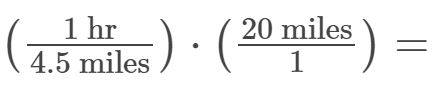# Rates

Rates typically are introduced to students in middle school and can be a challenging subject. Even though we use rates in everyday situations, such as miles per hour or miles per gallon, dealing with them in a formal manner is not always straightforward.

Example 1:

If John walks 2.25 miles in half an hour, what is his rate in miles per hour?

-Take 2.25 mi and divide it by 1/2 an hour. That gives use 4.5 miles per hour.

At this rate, how long would it take to travel 20 miles?

In order to answer this, first find unit rate. We’ve already found the unit rate of 4.5 mph. Now multiply 1 hr over 4.5 miles times 20 miles. By doing this we get the “miles” unit to cancel (a mile divided by a mile is 1) and we’re left with hours as our unit, which is what we want. In other words, we’ve divided 20 miles by 4.5 miles, which is probably common sense to most people but it’s important to do it this way so we can keep track of units as we get more complex problems.Our answer should be 4.44 hrs, which sounds reasonable.